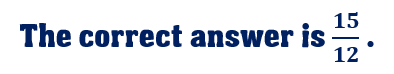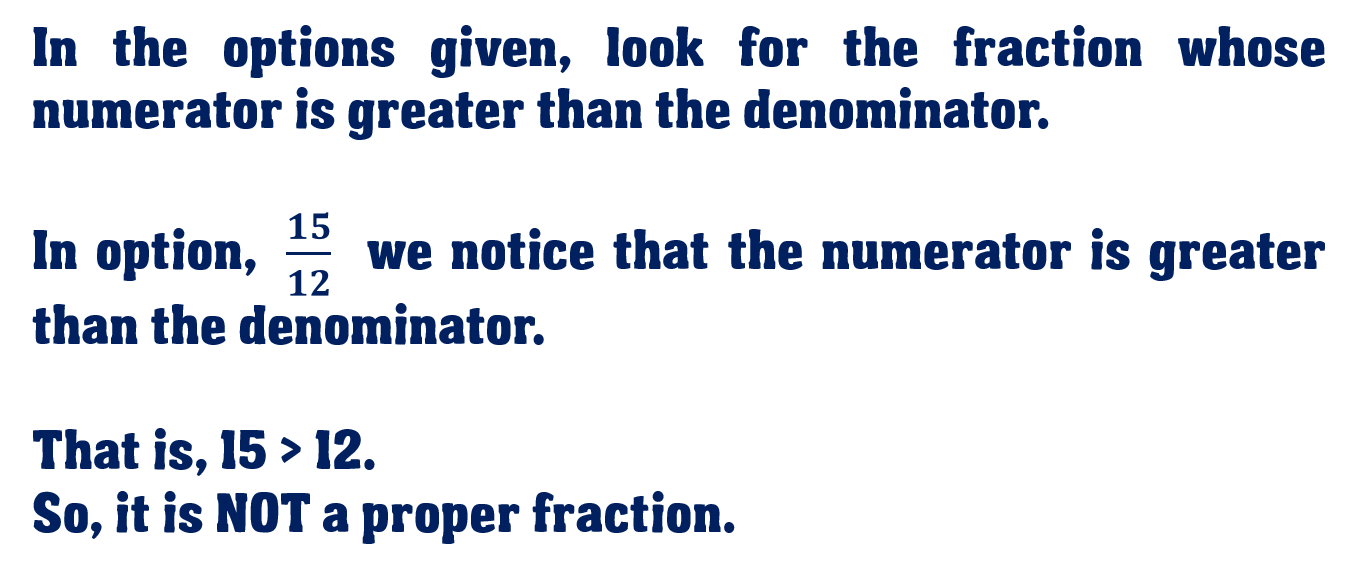1
visibility

Which one of the following is NOT a proper fraction?

• \$latex \dfrac{7}{10}\$

• \$latex \dfrac{16}{24}\$

• \$latex \dfrac{15}{12}\$

• \$latex \dfrac{14}{19}\$Please Note:

A proper fraction is a fraction whose numerator (the top number) is less than the denominator (the bottom number).

An improper fraction is a fraction whose numerator (the top number) is greater than the denominator (the bottom number).

Solution: